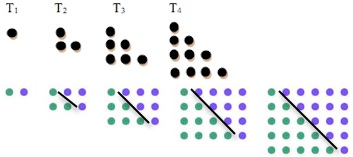Is a picture worth a thousand words (as the saying goes)?

This picture shows how you can fit 2 identical copies of a triangle together to form a rectangle.

Can you use it to find the number of dots in the fifth triangle number without counting the dots?

Can you find any triangle number in the same way?

Can you write down a formula for the nth triangle number $$T_n$$?

Now try this! Write out this expression for the ninth triangle number:

$$T_ 9 = 1 + 2 + 3 + 4 + 5 + 6 + 7 + 8 + 9$$

and then write it out again underneath but this time write it backwards:

$$T_ 9 = 9 + 8 + 7 + … + 1.$$

Add these two expressions together to find $$2\times T_9$$ and a formula for $$T_9$$.

Can you use the same method to find a formula for $$T_n$$? How does this method relate to the picture method?